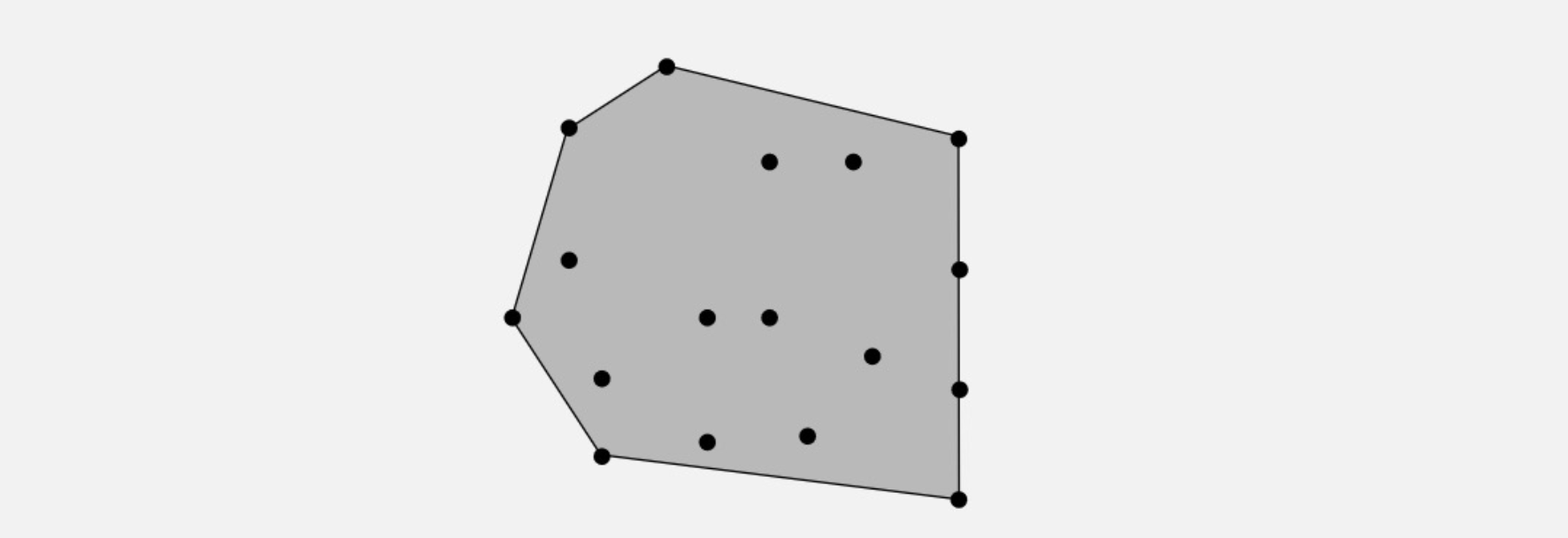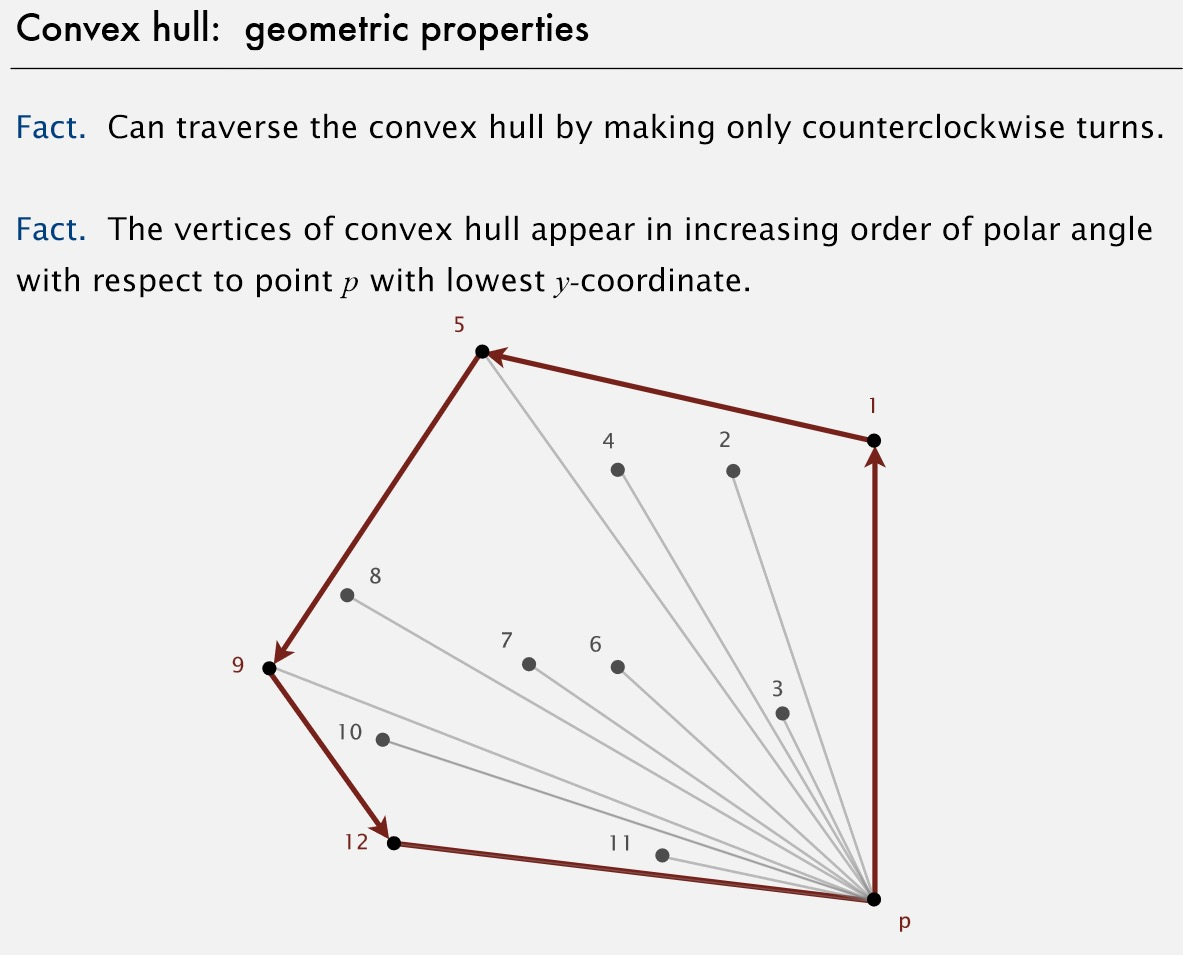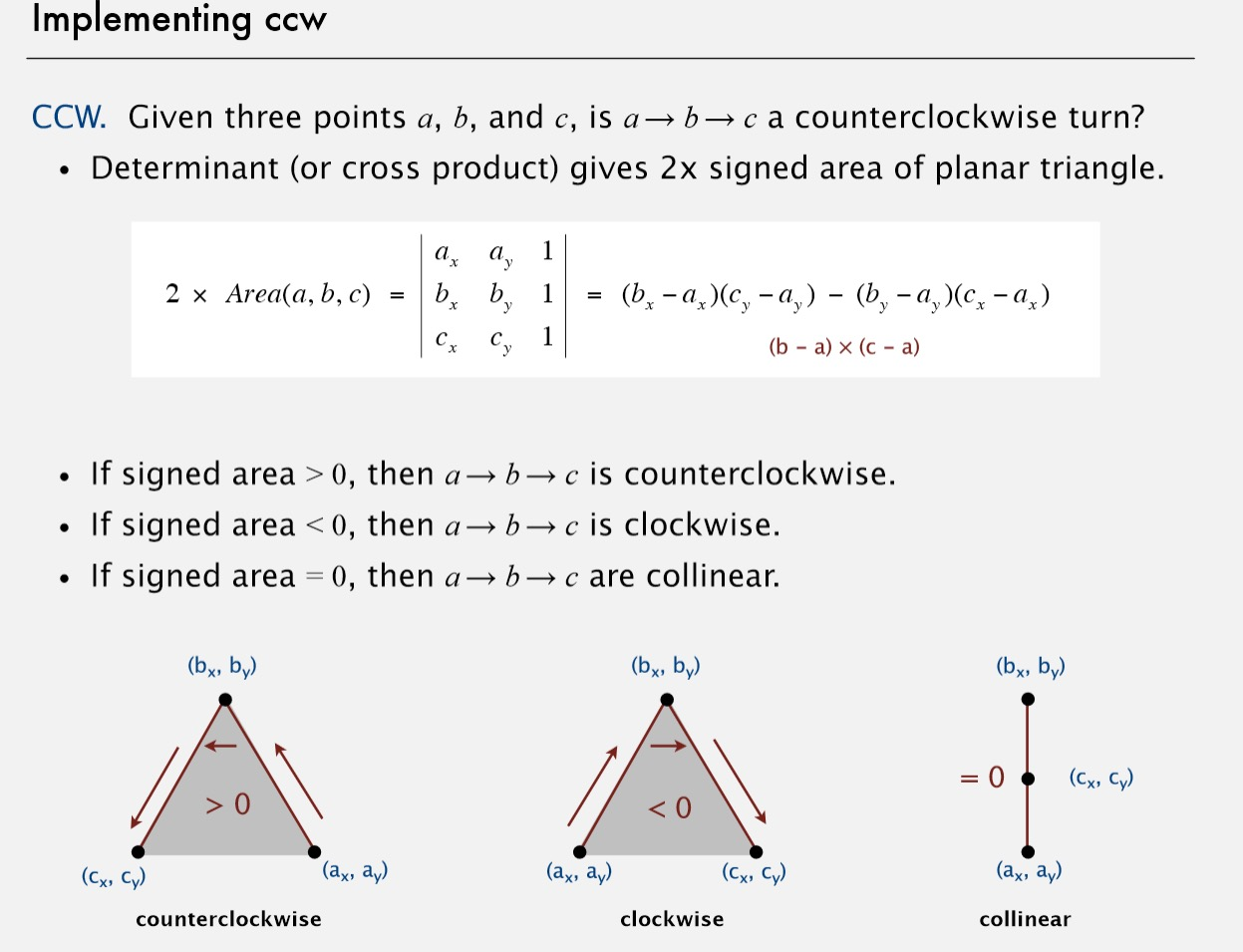# 元素排序(4)——凸包问题

2016-05-10   |     |     |

# 凸包问题## 凸包的几何性质

• 可以通过逆时针遍历所有凸包顶点
• 凸包的顶点是关于p的极角的递增序列，而p拥有最小的y坐标。## Graham 扫描算法

1. 选择y坐标最小的p
• 如何确定最小y坐标？
循环一遍
2. 对所有的点按关于p的 极角排序
• 如何对点进行极角排序？
• 如何效率的排序？
用MergeSort
3. 按顺序连接每个点，如果发现打破\$p_{i−2}→p_{i−1}→p_i\$ 打破了逆时针的原则，则舍弃\$p_{i−1},p_i\$。

• 如何判断是否打破了逆时针原则ccw？
• 如何解决退化问题（多个点在一条直线上）

通过计算（b-a）和（c-a）的叉乘就行了。
如果area > 0 则是逆时针，
如果area < 0 则是顺时针
如果area = 0 则是共线### 代码

``````public class Point2D
{
private final double x;
private final double y;
public Point2D(double x, double y)
{
this.x = x;
this.y = y;
}
...
public static int ccw(Point2D a, Point2D b, Point2D c)
{
double area2 = (b.x-a.x)*(c.y-a.y) - (b.y-a.y)*(c.x-a.x);
if      (area2 < 0) return -1;
// clockwise
else if (area2 > 0) return +1;
// counter-clockwise
else                return  0;
// collinear
}
}
stack<Point2D> hull = new Stack<Point>();
Array.sort(p,Point2D.Y_ORDER);//find p
Array.sort(p,p,BY_POLAR_ORDER)//

hull.push(p);
hull.push(p);

for(i = 2;i <　N;i++)
{
Point2D top = hull.pop();
// 如果是小于0，说明是顺时针，继续Pop一个
while(Point2D.ccw(hull.peek(),top,p[i]) <= 0)
top = hull.pop();
hull.push(top);
hull.push(p[i]);

}
``````